## RD Sharma Class 8 Solutions Chapter 21 Mensuration II Ex 21.2

These Solutions are part of RD Sharma Class 8 Solutions. Here we have given RD Sharma Class 8 Solutions Chapter 21 Mensuration II Ex 21.2

Other Exercises

Question 1.
Find the volume in cubic metres (cu.m) of each of the cuboids whose dimensions are :
(i) length = 12 cm, breadth = 10 m, height = 4.5 m
(ii) length = 4 m, breadth = 2.5 m, height = 50 cm
(iii) length = 10 m, breadth = 25 dm, height = 25 cm.
Solution:
(i) Length of cuboid (l) = 12 m
and height (h) = 4.5 m
∴Volume = l x b x h = 12 x 10 x 4.5 m3
= 540 m3
(ii) Length of cuboid (l) = 4 m
Height (h) = 50 cm = 0.5 m
∴ Volume = l x b x h = 4 x 2.5 x 0.5 = 5 m3
(iii) Length of cuboid (l) = 10 m
Breadth (b) = 25 dm = 2.5 m
Height (h) = 25 cm 0.25 m
∴ Volume = l x b x h = 10 x 2.5 x 0.25 m3 = 6.25 m3

Question 2.
Find the volume in cubic decimetre of each of the cubes whose side is
(i) 1.5 m
(it) 75 cm
(iii) 2 dm 5 cm
Solution:
(i) Side of cube (a) = 1.5 m
∴ Volume = a3 = (1.5)3 m3
= 1.5 x 1.5 x 1.5 m3 = 3.375 m3
= 3.375 x 1000 = 3375 dm3
(ii) Side of cube (a) = 75 cm = 7.5 dm
∴ Volume = a3 = (7.5)3 dm3
= 421.875 dm3
(iii) Side of cube (a) = 2 dm 5 cm = 2.5 dm
∴ Volume = (a)3 = (2.5)3 dm3
= 15.625 dm3

Question 3.
How much clay is dug out in digging a well measuring 3m by 2m by 5m?
Solution:
Length of well (l) = 3m
and height (depth) (h) = 5 m
Volume of earth dug out = l x b x h = 3 x 2 x 5 = 30m3

Question 4.
What will be the height of a cuboid of volume 168 m3, if the area of its base is 28 m2 ?
Solution:
Volume of a cuboid = 168 m3
Area of its base l.e., l x b = 28 m3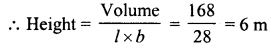Question 5.
A tank is 8 m long, 6 m broad and 2 m high. How much water can it contain ?
Solution:
Length of tank (l) = 8 m
Height (h) = 2 m
∴ Volume of water in the tank = l x b x h = 8 x 6 x 2 = 96 m3
= 96 x 1000 = 96000litres (∵1m3 = 1000litre)

Question 6.
The capacity of a certain cuboidal tank is 50000 litres of water. Find the breadth of the tank if its height and length are 10 m and 2.5 m respectively.
Solution:
Capacity of water in the tank = 50000 litres
∴ Volume of water = 50000 x $$\frac { 1 }{ 1000 }$$ = 50 m3 (1000 l = 1 m3)
Height of tank (h)= 10 m
and length (l) = 2.5 m
Volume 50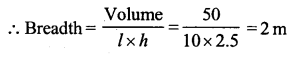Question 7.
A rectangular diesel tanker is 2 m long, 2 m wide and 40 cm deep. How many litres of diesel can it hold ?
Solution:
Length of tanker (l) = 2 m
Depth (h) = 40 cm = 0.4 m
∴ Volume = l x bx h = 2 x 2 x 0.4=1.6m3
Quantity of diesel = 1.6 x 1000 litres (1 m3= 1000 l)
= 1600 litres

Question 8.
The length, breadth and height of a room are 5 m, 4.5 m and 3 m, respectively. Find the volume of the air it contains.
Solution:
Length of room (l) = 5 m
and height (h) = 3 m
∴ Volume of air it contains
= l x b x h = 5 x 4.5 x 3 m3
= 67.5 m3

Question 9.
A water tank is 3 m long, 2 m broad and 1 m deep. How many litres of water can it hold ?
Solution:
Length of tank (l) = 3 m
and depth (h) = 1 m
∴ Volume of tank = l x b x h
= 3 x 2 x 1 = 6 m3
∴ Quantity of water it can contains
= 6 x 1000 litres = 6000 litres (1 m3= 1000 litres)

Question 10.
How many planks each of which is 3 m long, 15 cm broad and 5 cm thick can be prepared from a wooden block 6 m long, 75 cm broad and 45 cm thick ?
Solution:
Length of wooden block (l) = 6 m
Width (b) = 75 cm = 0.75 m
Thickness (h) = 45 cm = 0.45 m
∴ Volume = l x b x h = 6 x 0.75 x 0.45 m3
Length of plank (l) = 3 m
Breadth (b) = 15 cm = 0.15 m
Thickness (h) = 5 cm = 0.05 m
∴ Volume = 3 x 0.15 x 0.05 m3
Number of planks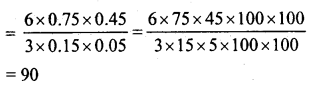Question 11.
How many bricks each of size 25 cm x 10 cm x 8 cm will be required to build a wall 5 m long, 3 m high and 16 cm thick assuming that the volume of sand and cement used in the construction is negligible ?
Solution:
Size of one brick = 25 cm x 10 cm x 8 cm
∴ Volume of one brick = 25 x 10 x 8 cm3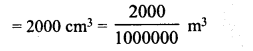Length of wall (l) = 5 m
Width (b) = 0.16 m
Height (h) = 3 m
∴ Volume of wall = l x b x h
= 5 x 0.16 x 3 m3 = 2.4 m3
∴ Number of bricks required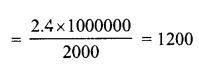Question 12.
A village, having a population of 4000 requires 150 litres water per head per day. It has a tank which is 20 m long, 15 m broad and 6 m high. For how many days the water of this tank will last ?
Solution:
Total population of a village = 4000
Water required for each person for one day = 150 litres
∴ Water required for 4000 persons for one day = 150 x 4000 = 600000 litres
Length of tank (l) = 20 m
Height (h) = 6 m
∴ Volume of tank = l x b x h = 20 x 15 x 6 m3 = 1800 m3
Capacity of water in the tank = 1800 x 1000 l= 1800000l (1 m3 = 1000 l)
∴ Number of days, the water will lastQuestion 13.
A rectangular field is 70 m long and 60 m broad. A well of dimensions 14 m x 8 m x 6 m is dug outside the field and the earth dugout from this well is spread evenly on the field. How much will the earth level rise ?
Solution:
Length of well (l) = 14 m
Depth (A) = 6m
∴ Volume of earth dugout = l x bx h
= 14 x 8 x 6 = 672 m3 Length of field = 70 m
Let h be the height of earth spread over
Then 70 x 60 x h = 672
⇒ h = $$\frac { 672 }{ 70×60 }$$ = 0.16m
∴ Height of earth = 0.16 m = 16 cm

Question 14.
A swimming pool is 250 m long and 130 m wide. 3250 cubic metres of water is pumped into it. Find the rise in the level of water.
Solution:
Volume of water = 3250 m3
Length of pool (l) = 250 m
∴ Height of water levelQuestion 15.
A beam 5 m long and 40 cm wide contains 0.6 cubic metres of wood. How thick is the beam?
Solution:
Volume of wood of the beam = 0.6 m3 = 600000
Length of beam (l) = 5 m = 500 cm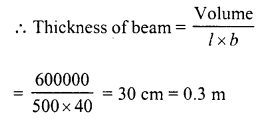Question 16.
The rainfall on a certain day was 6 cm. How many litres of water fell on 3 hectares of field on that day ?
Solution:
Area of the field = 3 hectares
= 3 x 10000 square metres
= 30000 square metres
Height of rainfall = 6 cm = m3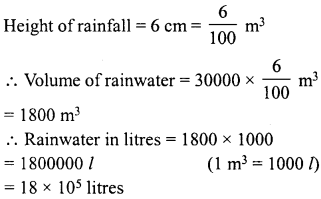Question 17.
An 8 m long cuboidal beam of wood when sliced produces four thousand 1 cm cubes and there is no wastage of wood in this process. If one edge of the beam is 0.5 m, find the third edge.
Solution:
Length of cuboidal beam (l) = 8 m = 800 cm
Number of cubical sliced = 4000
Edge of each cube = 1 cm
Volume of beam = 4000 (1)3 cm3 = 4000 cm3
One edge of the beam = 0.5 m = 50 cm.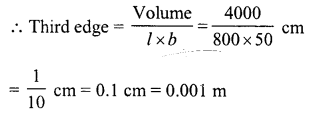Question 18.
The dimensions of a metal block are 2.25 m by 1.5 m by 27 cm. It is melted and recast into cubes, each of the side 45 cm. How many cubes are formed ?
Solution:
Dimensions of metal block = 2.25 m x 1.5 m x 27 cm
∴ Volume = 2.25 x 1.5 x 0.27 m3
= 225 x 150 x 27 cm3 = 911250 cm3
Side of each cube (a) = 45 cm
∴ Volume of one cube = a3 = (45)3 cm3 = 91125 cm3
∴ Number of cubes = $$\frac { 911250 }{ 91125 }$$ = 10

Question 19.
A solid rectangular piece of iron measures 6 m by 6 cm by 2 cm. Find the weight of this piece if 1 cm3 of iron weighs 8 gm.
Solution:
Dimensions of a piece of rectangular iron = 6m x 6cm x 2cm
∴ Volume = 600 x 6 x 2 cm3 = 7200 cm3
Weight of 1 cm3 = 8 gm
∴ Total weight of the piece = 7200 x 8 gm
= 57600 gm = $$\frac { 57600 }{ 1000 }$$ kg = 57.6 kg

Question 20.
Fill in the blanks in each of the following so as to make the statement true :
(i) 1 m3 = ……… cm3
(ii) 1 litre = …….. cubic decimetre
(iii) 1 kl = …… m3
(iv) The volume of a cube of side 8 cm is …….. .
(v) The volume of wooden cuboid of length 10 cm and breadth 8 cm is 4000 cm3. The height of the cuboid is …….. cm
(vi) 1 cu.dm = …….. cu.mm
(vii) 1 cu.km = ……cu.m
(viii) 1 litre =…….. cu.cm
(ix) 1 ml = ……… cu.cm
(x) 1 kl = ……… cu.dm = ……. cu.cm.
Solution:
(i) 1 m3 = 1000000 or 10cm3
(ii) 1 litre = 1 cubic decimetre
(iii) 1 kl = 1 m3
(iv) The volume of a cube of side 8 cm is 512 cm3 (V = a3 = 8 x 8 x 8 = 512 cm3)
(v) The volume of a wooden cuboid of length 10 cm and breadth 8 cm is 4000 cm3. The height of the cuboid is 50 cm(vi) 1 cu.dm = 1000000 cu mm = 106 cu.mm
(vii) 1 cu.km = 1000 x 1000 x 1000 cu.m = 109 cu.m
(viii) 1 litre = 1000 cu.cm = 103 cu.cm
(ix) 1 ml = 1 cu.cm
(x) 1 kl = 1000 cu.dm = 100 x 100 x 100 cu.cm = 106 cu.cm

Hope given RD Sharma Class 8 Solutions Chapter 21 Mensuration II Ex 21.2 are helpful to complete your math homework.

If you have any doubts, please comment below. Learn Insta try to provide online math tutoring for you.